1596689880

# Visualizing Filters and Feature Maps in Convolutional Neural Networks

When** dealing with image’s and image data, CNN are the go-to architectures. Convolutional neural networks have proved to provide many state-of-the-art solutions in deep learning and computer vision. Image recognition, object detection, self-driving cars would not be possible without CNN.

But when it comes down to how CNN see’s and recognize the image the way they do, things can be trickier.

• How a CNN decides whether a image is a cat or dog ?
• What makes a CNN more powerful than other models when it comes to image classification problems ?
• How and what do they see in an image ?

These were some of the questions i had back back when i first learned about CNN. The questions will grow as you deep dive into it.

Back then i heard about these terms filters and featuremaps, but dont know what they are and what they do. Later i know what they are but dont know what they look like but now, i know. When dealing with **Deep Convolutional Networks **filters and featuremaps are important. Filters are what makes the Featuremaps and that’s what the model see’s.

# What are Filters and FeatureMaps in CNN?

Filters** are set of weights which are learned using the backpropagation algorithm. If you do alot of practical deep learning coding, you may know them as kernels. Filter size can be of 3×3 or maybe 5×5 or maybe even 7×7.

Filters in a CNN layer learn to detect abstract concepts like boundary of a face, edges of a buildings etc. By stacking more and more CNN layers on top of each other, we can get more abstract and in-depth information from a CNN.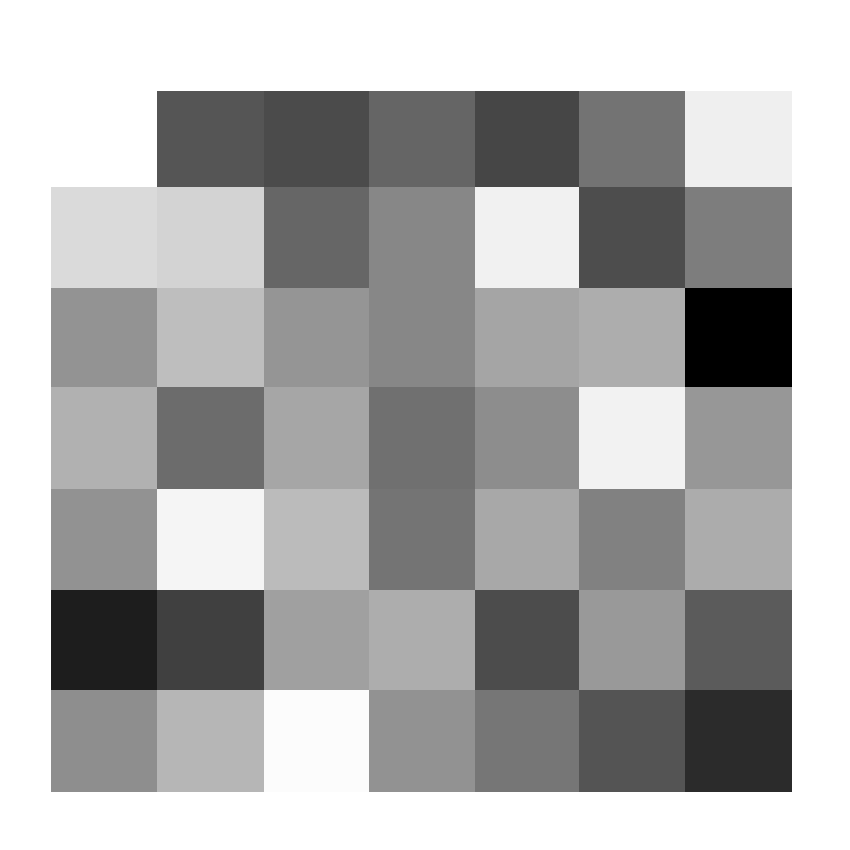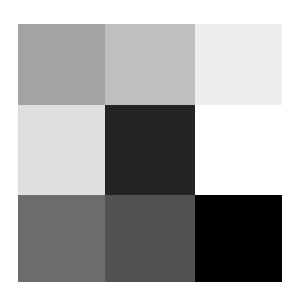7×7 and 3×3 filters

F

**eature Maps **are the results we get after applying the filter through the pixel value of the image.This is what the model see’s in a image and the process is called convolution operation. The reason for visualising the feature maps is to gain deeper understandings about CNN.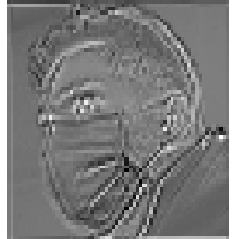Feature map

# Selecting the model

We will use the ResNet-50 neural network model for visualizing filters and feature maps. Using a ResNet-50 model for visualizing filters and feature maps is not ideal. The reason is that the resnet models in general, are a bit complex. Traversing through the inner convolutional layers can become quite difficult. You will learn how to access the inner convolutional layers of a difficult architecture. In the future, you will feel much more comfortable working with similar or more complex architectures.

The image i used is a photo from pexels. Its a image i collected to train my face-detection classifier.

#deep-learning #convolutional-network #python3 #pytorch #visualization #deep learning

## Buddha Community1596689880

## Visualizing Filters and Feature Maps in Convolutional Neural Networks

When** dealing with image’s and image data, CNN are the go-to architectures. Convolutional neural networks have proved to provide many state-of-the-art solutions in deep learning and computer vision. Image recognition, object detection, self-driving cars would not be possible without CNN.

But when it comes down to how CNN see’s and recognize the image the way they do, things can be trickier.

• How a CNN decides whether a image is a cat or dog ?
• What makes a CNN more powerful than other models when it comes to image classification problems ?
• How and what do they see in an image ?

These were some of the questions i had back back when i first learned about CNN. The questions will grow as you deep dive into it.

Back then i heard about these terms filters and featuremaps, but dont know what they are and what they do. Later i know what they are but dont know what they look like but now, i know. When dealing with **Deep Convolutional Networks **filters and featuremaps are important. Filters are what makes the Featuremaps and that’s what the model see’s.

# What are Filters and FeatureMaps in CNN?

Filters** are set of weights which are learned using the backpropagation algorithm. If you do alot of practical deep learning coding, you may know them as kernels. Filter size can be of 3×3 or maybe 5×5 or maybe even 7×7.

Filters in a CNN layer learn to detect abstract concepts like boundary of a face, edges of a buildings etc. By stacking more and more CNN layers on top of each other, we can get more abstract and in-depth information from a CNN.7×7 and 3×3 filters

F

**eature Maps **are the results we get after applying the filter through the pixel value of the image.This is what the model see’s in a image and the process is called convolution operation. The reason for visualising the feature maps is to gain deeper understandings about CNN.Feature map

# Selecting the model

We will use the ResNet-50 neural network model for visualizing filters and feature maps. Using a ResNet-50 model for visualizing filters and feature maps is not ideal. The reason is that the resnet models in general, are a bit complex. Traversing through the inner convolutional layers can become quite difficult. You will learn how to access the inner convolutional layers of a difficult architecture. In the future, you will feel much more comfortable working with similar or more complex architectures.

The image i used is a photo from pexels. Its a image i collected to train my face-detection classifier.

#deep-learning #convolutional-network #python3 #pytorch #visualization #deep learning1594925580

## Abstract

In this blog post, we present a formal treatment of receptive fields of convolution layers and characterizations of multi-scale convolutional feature maps using a derived mathematical framework. Using the developed mathematical framework, we compute the receptive fields and spatial scales of feature maps under different convolutional and pooling operations. We show the significance of pooling operations to ensure the exponential growth of spatial scale of feature maps as a function of layer depths. Also, we observe that without pooling operations embedded into CNNs, feature map spatial scales only grow linearly as layer depth increase. We introduce spatial scale profile as the layer-wise spatial scale characterization of CNNs which could be used to assess the compatibility of feature maps with histograms of object dimensions in training datasets. This use case is illustrated by computing the spatial scale profile of ResNet-50. Also, we explain how feature pyramid module generates multi-scale feature maps enriched with augmented semantic representations. Finally, it is shown while dilated convolutional filters preserve the spatial dimensions of feature maps, they maintain greater exponential growth rate of spatial scales compared to their regular convolutional filter counterparts.

_Reading this blogpost, you will have a deeper insight into the intuitions behind the use cases of multi-scale convolutional feature maps in the recent proposed CNN architectures for variety of vision tasks. Therefore, this blogpost can be treated as a tutorial to learn more about how different types of layers impact the spatial scales and receptive fields of feature maps. Also, this blogpost is for those engineers and researchers that are involved in designing CNN architectures and are tired of blind trial and error of which feature maps to choose from a CNN backbone to improve the performance of their models, and instead, prefer from the early steps of design process, to match the spatial scale profiles of feature maps with the object dimensions in training datasets. To facilitate such use cases, we have made our code base publicly available at _https://github.com/rezasanatkar/cnn_spatial_scale.

## Introduction

It is a general assumption and understanding that feature maps generated by the early convolutional layers of CNNs encode basic semantic representations such as edges and corners, whereas deeper convolutional layers encode more complex semantic representations such as complicated geometric shapes in their output feature maps. Such a characteristic of CNNs to generate feature maps with multi semantic levels is resultant of their hierarchical representational learning ability which is based on multi-layer deep structures. Feature maps with different semantic levels are critical for CNNs because of the two following reasons: (1) complex semantics feature maps are built on top of basic semantic feature maps as their building blocks (2) a number of vision tasks like instance and semantic segmentation benefit from both basic and complex semantic feature maps. A vision CNN-based architecture takes an image as input, and passes it through several convolutional layers with the goal of generating semantic representations corresponding to the input image. In particular, each convolution layer outputs a feature map, where the extent of the encoded semantics in that feature map depends on both the representational learning ability of that convolutional layer as well as its previous convolutional layers.

## CNN Feature Maps are Spatial Variance

One important characteristic of CNN feature maps is that they are spatial variance, meaning that CNN feature maps have spatial dimensions, and a feature encoded by a given feature map might only become active for a subset of spatial regions of the feature map. In order to better understand the spatial variance property of CNN feature maps, first, we need to understand why the feature maps generated by fully connected layers are not spatial variance. The feature maps generated by fully connected layers (you can thinks of the activations of neurons of a given fully connected layer as its output feature map) do not have spatial dimensions since every neuron of a fully connected layer is connected to all the input units of the fully connected layer. Therefore, it is not possible to define and consider a spatial aspect for a neuron activation output.

On the other hand, every activation of a CNN feature map is only connected to a few input units, which are in each other spatial neighborhood. This property of CNN feature maps gives rise to their spatial variance characteristic, and is resultant from the spatial local structure of convolution filters and their spatially limited receptive fields. The differences between fully connected layers and convolutional layers which result in spatial invariance for one and spatial variance for the other one is illustrated in the below image where the input image is denoted by the green rectangle, and the brown rectangle denotes a convolutional feature map. Also, a fully connected layer with two output neurons is denoted by two blue and grey circles. As you can see, each neuron of the fully connected layer is impacted by all the image pixels whereas each entry of feature map is only impacted by a local neighborhood of input pixels.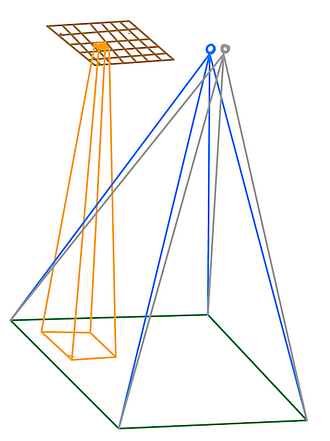This figure illustrates why the features generated by fully connected layers are not spatial variance while convolutional layers generate spatial variance feature maps. The green rectangle denotes the input image and the brown rectangle denotes a feature map with dimension 5 x 7 x 1 generated by a convolution layer of a CNN. On the other hand, the two blue and grey circles denote activation outputs of a fully connected layer with two output neurons. Let assume the blue neuron (feature) of the fully connected layer will become active if there is a bicycle in the input image while its grey neuron (feature) will become active if there is car in the input image. In other words, the blue neuron is the bicycle feature while the grey neuron is the car feature. Because of the nature of fully connected layers which each neuron’s output is impacted by all the input image pixels, the fully connected layers’ generated features cannot encode any localization information out-of-the-box in order to tell us where in the input image the bicycle is located if there is a bicycle in input image. On the other hand, the feature maps generated by convolutional layers are spatial variance and therefore, they encode the localization information in addition to the existence information of objects. In particular, a generated feature map of dimension W x H x C by a convolutional layer, contains information of existence of C different features (each channel, the third dimension of the feature map, encode existence information of a unique feature) where the spatial dimension W x H of features tell us for which location of the input image, the feature is activated. In this example, the brown convolutional feature map only encodes one feature since it has only one channel (its third dimension is equal to one). Assuming this brown feature map is the bicycle feature map, then an entry of this feature map becomes active only if there is a bicycle in the receptive field of that entry in input image. In other words, this entry does not become active if there is a bicycle in the input image but not in its specific receptive field. Such property of convolutional feature maps enable them not only to encode information about the existence of objects in input images, but also to encode localization information of objects.

#convolutional-network #dilated-convolution #receptive-field #feature-pyramid-network #neural networks1596826500

## Convolutional Neural Networks-An Intuitive approach

A simple yet comprehensive approach to the concepts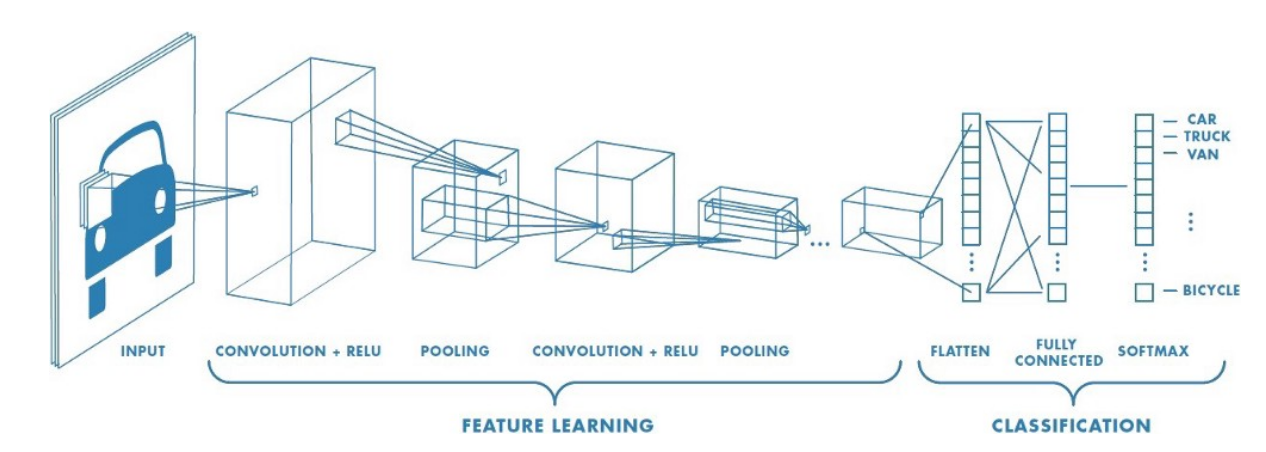Convolutional Neural Networks

Artificial intelligence has seen a tremendous growth over the last few years, The gap between machines and humans is slowly but steadily decreasing. One important difference between humans and machines is (or rather was!) with regards to human’s perception of images and sound.How do we train a machine to recognize images and sound as we do?

At this point we can ask ourselves a few questions!!!

How would the machines perceive images and sound ?

How would the machines be able to differentiate between different images for example say between a cat and a dog?

Can machines identify and differentiate between different human beings for example lets say differentiate a male from a female or identify Leonardo Di Caprio or Brad Pitt by just feeding their images to it?

Let’s attempt to find out!!!

The Colour coding system:

Lets get a basic idea of what the colour coding system for machines is

RGB decimal system: It is denoted as rgb(255, 0, 0). It consists of three channels representing RED , BLUE and GREEN respectively . RGB defines how much red, green or blue value you’d like to have displayed in a decimal value somewhere between 0, which is no representation of the color, and 255, the highest possible concentration of the color. So, in the example rgb(255, 0, 0), we’d get a very bright red. If we wanted all green, our RGB would be rgb(0, 255, 0). For a simple blue, it would be rgb(0, 0, 255).As we know all colours can be obtained as a combination of Red , Green and Blue , we can obtain the coding for any colour we want.

Gray scale: Gray scale consists of just 1 channel (0 to 255)with 0 representing black and 255 representing white. The colors in between represent the different shades of Gray.

Computers ‘see’ in a different way than we do. Their world consists of only numbers.

Every image can be represented as 2-dimensional arrays of numbers, known as pixels.

But the fact that they perceive images in a different way, doesn’t mean we can’t train them to recognize patterns, like we do. We just have to think of what an image is in a different way.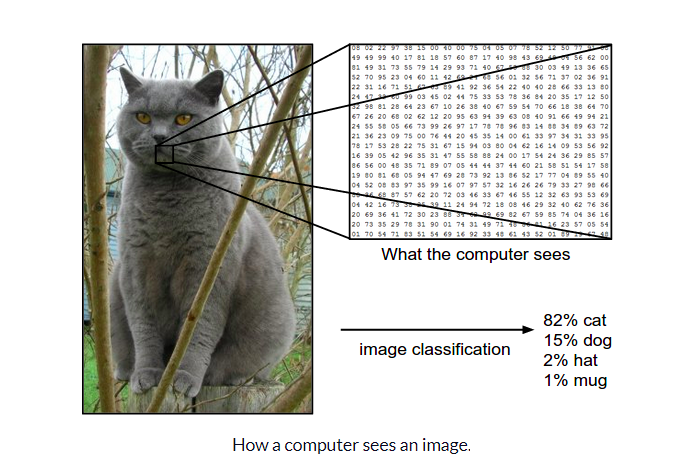Now that we have a basic idea of how images can be represented , let us try and understand The architecture of a CNN

CNN architecture

Convolutional Neural Networks have a different architecture than regular Neural Networks. Regular Neural Networks transform an input by putting it through a series of hidden layers. Every layer is made up of a set of neurons, where each layer is fully connected to all neurons in the layer before. Finally, there is a last fully-connected layer — the output layer — that represent the predictions.

Convolutional Neural Networks are a bit different. First of all, the layers are organised in 3 dimensions: width, height and depth. Further, the neurons in one layer do not connect to all the neurons in the next layer but only to a small region of it. Lastly, the final output will be reduced to a single vector of probability scores, organized along the depth dimension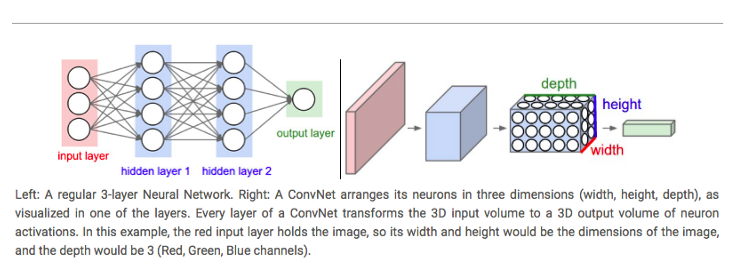A typical CNN architecture

As can be seen above CNNs have two components:

• The Hidden layers/Feature extraction part

In this part, the network will perform a series of **convolutions **and pooling operations during which the features are detected. If you had a picture of a tiger , this is the part where the network would recognize the stripes , 4 legs , 2 eyes , one nose , distinctive orange colour etc.

• The Classification part

Here, the fully connected layers will serve as a classifier on top of these extracted features. They will assign a** probability** for the object on the image being what the algorithm predicts it is.

Before we proceed any further we need to understand what is “convolution”, we will come back to the architecture later:

What do we mean by the “convolution” in Convolutional Neural Networks?

Let us decode!!!

#convolutional-neural-net #convolution #computer-vision #neural networks1596825840

## A Comparative Analysis of Recurrent Neural Networks

Recurrent neural networks, also known as RNNs, are a class of neural networks that allow previous outputs to be used as inputs while having hidden states. RNN models are mostly used in the fields of natural language processing and speech recognition.

The vanishing and exploding gradient phenomena are often encountered in the context of RNNs. The reason why they happen is that it is difficult to capture long term dependencies because of multiplicative gradient that can be exponentially decreasing/increasing with respect to the number of layers.

Gated Recurrent Unit (GRU) and Long Short-Term Memory units (LSTM) deal with the vanishing gradient problem encountered by traditional RNNs, with LSTM being a generalization of GRU.

1D Convolution_ layer_ creates a convolution kernel that is convolved with the layer input over a single spatial (or temporal) dimension to produce a tensor of outputs. It is very effective for deriving features from a fixed-length segment of the overall dataset. A 1D CNN works well for natural language processing (NLP).

## DATASET: IMDb Movie Review

TensorFlow Datasets is a collection of datasets ready to use, with TensorFlow or other Python ML frameworks, such as Jax. All datasets are exposed as `[_tf.data.Datasets_](https://www.tensorflow.org/api_docs/python/tf/data/Dataset)`, enabling easy-to-use and high-performance input pipelines.

“imdb_reviews”

This is a dataset for binary sentiment classification containing substantially more data than previous benchmark datasets. It provides a set of 25,000 highly polar movie reviews for training, and 25,000 for testing.

## Import Libraries

``````import pandas as pd
import numpy as np
import matplotlib.pyplot as plt
import seaborn as sns
%matplotlib inline
``````

``````import tensorflow as tf
import tensorflow_datasets

imdb
``````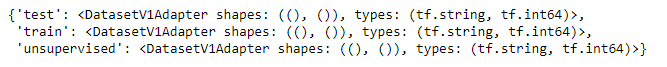``````info
``````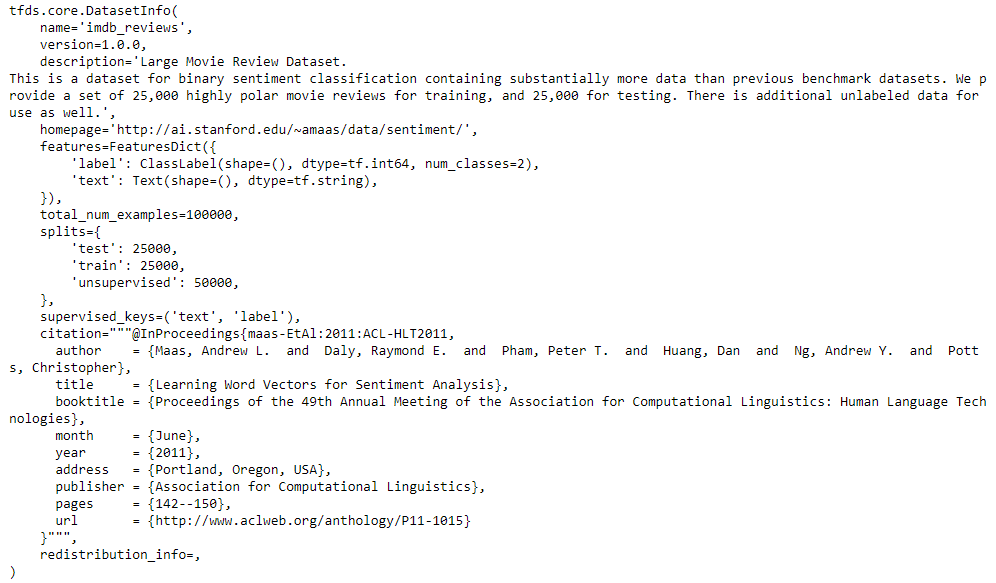## Training and Testing Data

``````train_data, test_data=imdb['train'], imdb['test']

training_sentences=[]
training_label=[]
testing_sentences=[]
testing_label=[]
for s,l in train_data:
training_sentences.append(str(s.numpy()))
training_label.append(l.numpy())
for s,l in test_data:
testing_sentences.append(str(s.numpy()))
testing_label.append(l.numpy())
training_label_final=np.array(training_label)
testing_label_final=np.array(testing_label)
``````

``````vocab_size=10000
embedding_dim=16
max_length=120
trunc_type='post'
oov_tok='<oov>'
from tensorflow.keras.preprocessing.text import Tokenizer
tokenizer= Tokenizer(num_words=vocab_size, oov_token=oov_tok)
tokenizer.fit_on_texts(training_sentences)
word_index=tokenizer.word_index
sequences=tokenizer.texts_to_sequences(training_sentences)
testing_sequences=tokenizer.texts_to_sequences(testing_sentences)
from tensorflow.keras.models import Sequential
from tensorflow.keras.layers import Dense, Dropout, Embedding
``````

## Multi-layer Bidirectional LSTM

#imdb #convolutional-network #long-short-term-memory #recurrent-neural-network #gated-recurrent-unit #neural networks1595691960

## How Graph Convolutional Networks (GCN) Work

In this post, we’re gonna take a close look at one of the well-known Graph neural networks named GCN. First, we’ll get the intuition to see how it works, then we’ll go deeper into the maths behind it.

## Why Graphs?

Many problems are graphs in true nature. In our world, we see many data are graphs, such as molecules, social networks, and paper citations networks.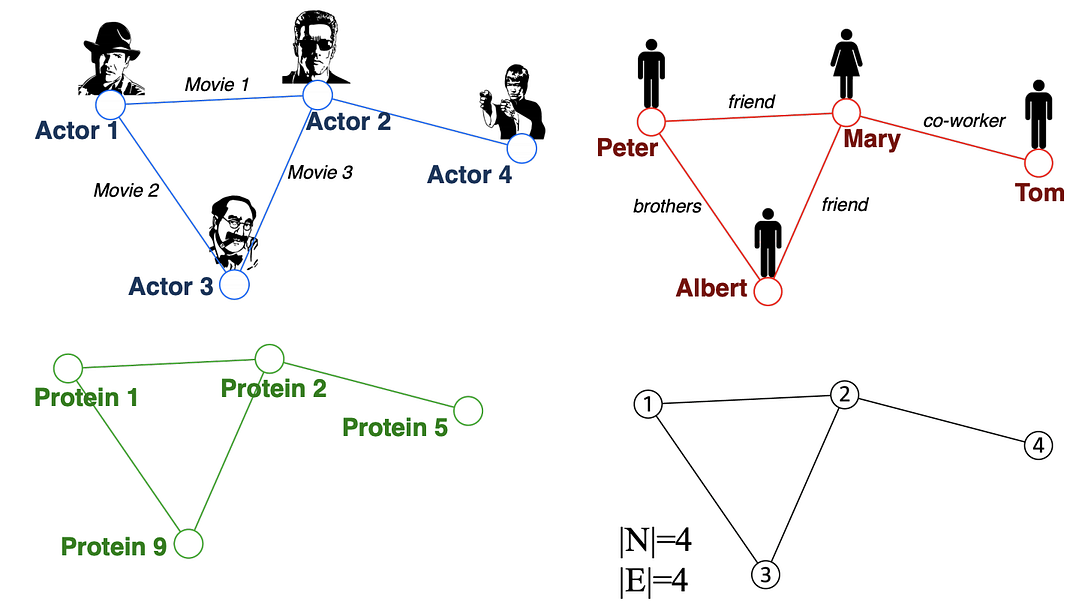Examples of graphs. (Picture from )

• Node classification: Predict a type of a given node
• Community detection: Identify densely linked clusters of nodes
• Network similarity: How similar are two (sub)networks

## Machine Learning Lifecycle

In the graph, we have node features (the data of nodes) and the structure of the graph (how nodes are connected).

For the former, we can easily get the data from each node. But when it comes to the structure, it is not trivial to extract useful information from it. For example, if 2 nodes are close to one another, should we treat them differently to other pairs? How about high and low degree nodes? In fact, each specific task can consume a lot of time and effort just for Feature Engineering, i.e., to distill the structure into our features.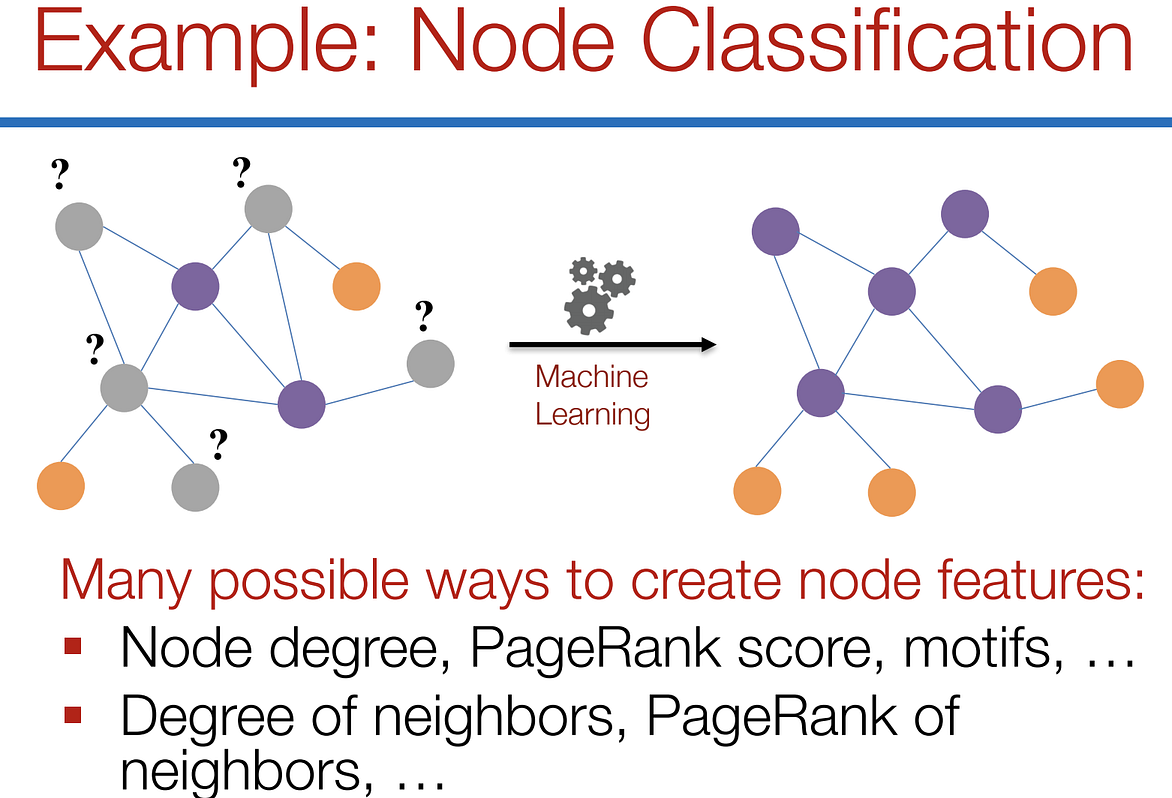Feature engineering on graphs. (Picture from )

It would be much better to somehow get both the node features and the structure as the input, and let the machine to figure out what information is useful by itself.

That’s why we need Graph Representation Learning.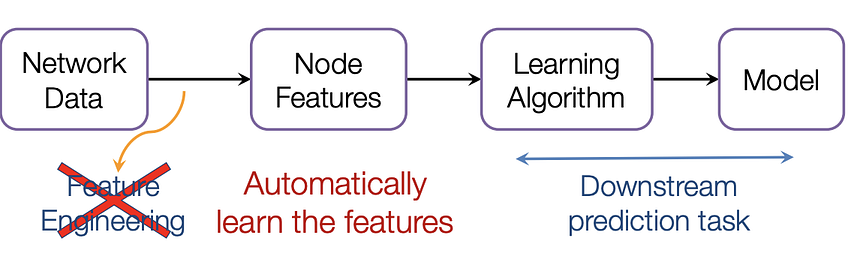We want the graph can learn the “feature engineering” by itself. (Picture from )

# Graph Convolutional Networks (GCNs)

Paper: Semi-supervised Classification with Graph Convolutional Networks(2017) 

GCN is a type of convolutional neural network that can work directly on graphs and take advantage of their structural information.

it solves the problem of classifying nodes (such as documents) in a graph (such as a citation network), where labels are only available for a small subset of nodes (semi-supervised learning).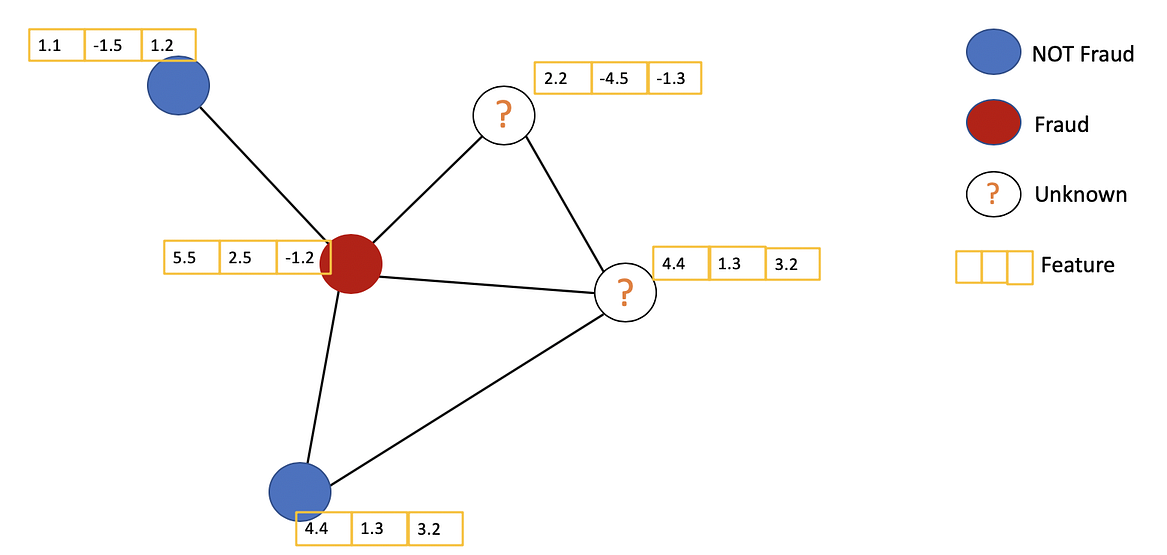Example of Semi-supervised learning on Graphs. Some nodes dont have labels (unknown nodes).

#graph-neural-networks #graph-convolution-network #deep-learning #neural-networks